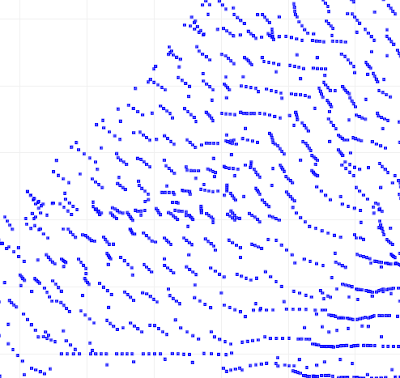## Tuesday, 15 June 2010

### Improving DoubleDouble performance with self-modifying methods

In a previous post I discussed how the DoubleDouble extended-precision API provides more robust computation for the Delaunay inCircle test. Quite rightly there were questions about the performance impact of using DoubleDouble. Of course, there is a performance penalty, but it's not as large as you might think, since the inCircle computation is only a portion of the cost of the overall Delaunay algorithm . For larger datasets the performance penalty is about 2x - which seems like an acceptable tradeoff for obtaining robust computation.

But after a bit of thought I realized that there was a simple way to improve the performance of using DoubleDouble. I originally designed the DoubleDouble API to provide value semantics, since this is a 100% safe way of evaluating expressions. However, this requires creating a new object to contain the results of every operation. The alternative is to provide self-modifying methods, which update the value of the object the method is called on. In many arithmetic expressions, it's possible to use self-methods for most operations. This avoids a lot of object instantiation and provides a significant performance benefit (even with Java's ultra-efficient object allocation code).

I added self-method versions of all the basic operations to the DoubleDouble API. While I was at it I threw in operations which took double arguments as well, since this is a common use case and allows avoiding even more allocations (as well as simplifying the code). The self-methods are all prefixed with the word "self", to make them stand out since they are potentially dangerous if used incorrectly. (I suppose a more Java-esque term would be "this-methods", but in this case the Smalltalk argot seems more elegant).

I also decided to rename the class to DD, to improve the readability of the code. Another enhancement might be to shorten the method names to 3 characters (e.g. "mul" instead of "multiply").

As an example, here's the inCircle code in the original implementation and using the improved API. Note the use of the new DD.sqr(double) function to improve readability as well as performance.

Original code
`public static boolean isInCircleDDSlow( Coordinate a, Coordinate b, Coordinate c, Coordinate p) {DD px = DD.valueOf(p.x);DD py = DD.valueOf(p.y);DD ax = DD.valueOf(a.x);DD ay = DD.valueOf(a.y);DD bx = DD.valueOf(b.x);DD by = DD.valueOf(b.y);DD cx = DD.valueOf(c.x);DD cy = DD.valueOf(c.y);DD aTerm = (ax.multiply(ax).add(ay.multiply(ay)))   .multiply(triAreaDDSlow(bx, by, cx, cy, px, py));DD bTerm = (bx.multiply(bx).add(by.multiply(by)))   .multiply(triAreaDDSlow(ax, ay, cx, cy, px, py));DD cTerm = (cx.multiply(cx).add(cy.multiply(cy)))   .multiply(triAreaDDSlow(ax, ay, bx, by, px, py));DD pTerm = (px.multiply(px).add(py.multiply(py)))   .multiply(triAreaDDSlow(ax, ay, bx, by, cx, cy));DD sum = aTerm.subtract(bTerm).add(cTerm).subtract(pTerm);boolean isInCircle = sum.doubleValue() > 0;return isInCircle;}`

Improved code
`public static boolean isInCircleDDFast( Coordinate a, Coordinate b, Coordinate c, Coordinate p) {DD aTerm = (DD.sqr(a.x).selfAdd(DD.sqr(a.y)))   .selfMultiply(triAreaDDFast(b, c, p));DD bTerm = (DD.sqr(b.x).selfAdd(DD.sqr(b.y)))   .selfMultiply(triAreaDDFast(a, c, p));DD cTerm = (DD.sqr(c.x).selfAdd(DD.sqr(c.y)))   .selfMultiply(triAreaDDFast(a, b, p));DD pTerm = (DD.sqr(p.x).selfAdd(DD.sqr(p.y)))   .selfMultiply(triAreaDDFast(a, b, c));DD sum = aTerm.selfSubtract(bTerm).selfAdd(cTerm).selfSubtract(pTerm);boolean isInCircle = sum.doubleValue() > 0;return isInCircle;}`

The table below summarizes the performance increase provided by using self-methods for inCircle. The penalty for using DD for improved robustness is now down to only about 1.5x slowdown.

Timings for Delaunay triangulation using inCircle predicate implemented using double-precision (DP), DoubleDouble (DD), and DoubleDouble with in-place operations (DD-self). All times in milliseconds.

# ptsDPDD-selfDD
1,000304780
10,000334782984
10,00079531257816000

## Wednesday, 9 June 2010

### Improvements to robustness in JTS Delaunay Triangulation

JTS 1.11 added the capability to compute Delaunay Triangulations of point sets. Although the triangulation code was extensively tested and used prior to release, it unfortunately didn't take long before someone uncovered a robustness issue. The problem occurred in a large dataset of over 288,000 points. When the JTS 1.11 Delaunay algorithm was run against this data, a TopologyException was thrown, preventing the computation from completing.

Here's a portion of the dataset which produced the problem:Digging into the code , it turned out (not unexpectedly) to be caused by a robustness failure in the inCircle predicate. InCircle is a key test used in Delaunay triangulation. The standard algorithm for computing it involves determining the sign of the determinant of a 4x4 matrix.This computation is notoriously prone to robustness failure when evaluated using double-precision arithmetic. The rounding errors inherent in using double-precision can cause the sign of the determinant to be computed incorrectly. (For an good explanation of the issue see this page by Jonathan Shewchuk.)

As a concrete example, consider testing whether the point

POINT (687958.13 7460720.99)

lies in the circumcircle of the triangle

POLYGON ((687958.05 7460725.97, 687957.43 7460725.93, 687957.58 7460721, 687958.05 7460725.97)

Visually this looks like:Zooming in, you can see that the query point is NOT in the circumcircle, so that InCircle()=FALSE.However, evaluating the inCircle predicate determinant using double-precision computes InCircle()=TRUE. This is because the large magnitude of the ordinate values relative to the size of the triangle causes the significant information to be lost in the roundoff error. The effect of this error propagates through the Delaunay algorithm. Ultimately this results in a convergence failure which is detected and reported.

Luckily, a solution was immediately to hand, in the form of the DoubleDouble extended-precision library I ported to Java a while ago. Re-implementing the predicate evaluation using DoubleDouble computed the correct results for inCircle in all cases. This eliminated the robustness failure, and allowed the Delaunay Triangulation of the entire 288K point dataset to be computed without errors (for the record, this took 5 min 20 s).The DoubleDouble code had been a solution in search of a problem for quite a while, so I was happy to get to prove its usefulness in a real-world situation.

And the story doesn't end there - it gets even better...